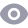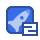### c语言指针练习0收藏

4)编写一个程序实现以下功能:用指针数组处理一个二维数组,要求求出二维数组所有元 素的和。 nt array4]={{7 14,30,3,·15} 0,5,27,·7} #ⅰnc|ud<sti0.h> nt mai n() i nt arr ay4]={{7,10,2,3:,114,30,6,·15}.10.5,27,·7)} i nt oa=arraylo, array[1l, array[211, i, i i nt s jm=0 or for(」=0:j<4;j++,pa[i++) sJm+=pa[i] pr f('% n, su m): return o 己知数组a[101和b10]中元泰的值遍增有序,指针实现将两个数组中的元素按递增 的顺序输出。 # i nal ude<stdi。.h> n!2[10={1,4,12,13,14,20,25,32,33,40)}; nb=[0,3.15,18,19,25,39,43,44,50]; whiIe(ip<(a+10)}&&(q<(b+101)) intf("%":‘p; 0++ el se print(% d,'91 0==(3+10)) eI se while( p <(+10) pri ntf( id,p++): printf(\ n": rei ur n 0 6)定义字符指针数组char*st5分别指向5个字符串常量,从小到大输出字符中的内容 finc ude <si o h> nt mai n( I,, ml n char str[5=" Shanghai Bei ng Guangz hou an Chongqin cha r{i=0 0r(j=i+1:j<5;j+-) i f( strcmp(str[min], str[j]>0 m n p= sir[i: . i]=st r[ mi n stri mi n]= p fo'(I=C:|<5;|++) pr("gsin, str[ili r et ur n 0: 7)已知数组内容如下s[]={1,2,3,4,5,6,7,8.9},输入一个数n(1<=n<=9),使得该数 组内容顺序后移n个位置。如n-3时,数组后移3个位置后的内容为7,8,9,1,2,3,4,5,5} #i nclude <stdio. h> #include <st dl ib. h> adeline len 9 nt maI n i nt ar r ay LEn=(1,2,3,4,5,6,7,E,9} num, t e mp, P print f( Pl ease input t he number(1.9 of el ament s you want t o move: "y scanf("n”,Bnum) f((um<1)(num>9) rinf( The nu mber is out of r ange 1-9) n exit(C1: Ior(=0; i <nu m; i++) t a mp ='I arr ay+LEN-1) f or( p=ar r ay+LEN- 1; p>arr ay; p-) mp. or(D=arr ay: p<array+LEN;p++ printf ("d",p) print f(V 8)输入一个字符串,内有数字和非数字字符,如a123×45617960?302ta5876将其中 连续的数宇作为一个整数,依次存放到整型数组a中。例如,123放在a,456放在a1], 统计共有多少个整数,并输出这些数。 # ncl ud彐<st0.h define len bo i nt mai 1() har str[lEN],p t arr ay LEN/ 21, val ue=0 int begin count =0 print f( Pl ease input a stri ng whi ch i uci ng any di gils: in: get s(st r) I pri nt f( %s\ whi l a( 'p 是&1‘p<='8')) begin_count 1 alue= val ue’10+p·"0 e se array[ n++=val ue begin- count =0 i f (begin_count ==1)array[++=val ue; f or(val ue=0; val e<n; val ve++) printf("%d", array value]) print f(l": I n 0:

...展开详情

• 5
资源
• 1
粉丝
•等级

最新推荐c语言指针练习 50积分/C币 立即下载
1/7试读结束， 可继续读1页

50积分/C币 立即下载 ＞